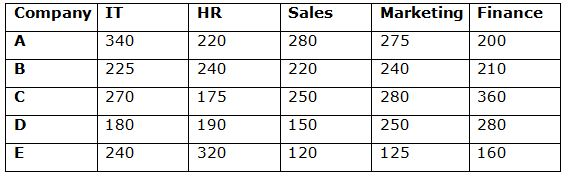# IBPS PO Prelims Quantitative Aptitude Questions 2019 (Day-16)

Dear Aspirants, Our IBPS Guide team is providing new series of Quantitative Aptitude Questions for IBPS PO Prelims 2019 so the aspirants can practice it on a daily basis. These questions are framed by our skilled experts after understanding your needs thoroughly. Aspirants can practice these new series questions daily to familiarize with the exact exam pattern and make your preparation effective.

Check here for IBPS PO Prelims Mock Test 2019

##### Check here for IBPS PO  Mock Test 2019
[WpProQuiz 7217]

Data Interpretation

Directions (1 – 5): Study the following information carefully and answer the questions given below.

The given table shows the number of employee works in different departments in different companies.1) What is the difference between the number of employee works in Sales and Finance departments in A, C and E together?

a) 50

b) 60

c) 70

d) 80

e) 40

2) What is the ratio of the number of employees in B to D in all the departments together?

a) 227: 219

b) 223: 210

c) 217: 205

d) 227: 210

e) None of these

3) The average number of employees in IT department in all the companies together is approximately what percent of the average Marketing department in all the companies together?

a) 103%

b) 107%

c) 111%

d) 113%

e) 99%

4) What is the difference between the total number of HR employees and Sales department employees in all the companies together?

a) 120

b) 125

c) 134

d) 179

e) None of these

5) What is the ratio of the average number of fiancé department employees in B and D together to the average number of HR employees in B, C and E together?

a) 1: 1

b) 2: 1

c) 1: 3

d) 2: 3

e) 3: 5

Application sums

6) A train crosses 200 m long platform in 15 seconds and also it crosses a man running same direction at the speed of 15kmph in 4 seconds. Find the length of train.

a) 100 m

b) 150 m

c) 200 m

d) 250 m

e) None of these

7) The ratio of the length to breadth of the rectangle is 4: 3 and the side of the square is 14 cm. If the perimeter of the square is equal to the perimeter of the rectangle, then what is the area of the rectangle?

a) 132 cm2

b) 144 cm2

c) 178 cm2

d) 192 cm2

e) None of these

8) Ratio of the number of male to female employees in the company is 3: 4. If the number of male and female employees increased by 42 and 36 respectively, then the ratio of the number of male to female of the company becomes 4: 5. What is the initial number of male employees in the company?

a) 198

b) 177

c) 168

d) 186

e) None of these

9) Rahul invests Rs.5000 in simple interest scheme at the rate of x% per annum for 5 years and Sam invests same amount in another simple interest scheme at the rate of (x + 5)% per annum for 3 years. If the difference between the interest received by Sam and Rahul is Rs.250, then find the value of x.

a) 5%

b) 8%

c) 10%

d) 15%

e) None of these

10) A, B and C complete a work in 8 days, 24 days and 12 days respectively. A and B started together and C joined them 2 days before the completion of the work. In how many days the work will be completed?

a) 7 days

b) 8 days

c) 6 days

d) 5 days

e) None of these

Directions (1-5) :

Number of sales employees in A, C and E = 280 + 250 + 120 = 650

Number of finance department employees in A, C and E = 200 + 360 + 160 = 720

Difference = 720 – 650 = 70

Required ratio = (225 + 240 + 220 + 240 + 210): (180 + 190 + 150 + 250 + 280)

= 1135: 1050

= 227: 210

IT department employees = (340 + 225 + 270 + 180 + 240)/5 = 1255/5 = 251

Marketing department employees = (275 + 240 + 280 + 250 + 125)/5 = 234

Required percentage = 251/234 * 100 = 107%

HR employees = 220 + 240 + 175 + 190 + 320 = 1145

Sales employees = 280 + 220 + 250 + 150 + 120 = 1020

Difference = 1145 – 1020 = 125

Required ratio = (210 + 280)/2: (240 + 175 + 320)/3

= 245: 245

= 1: 1

Directions (6-10) :

Let Length of train = x

Speed of train = y

200 + x = y * 5/18 * 15

1200 + 6x = 25y ———-(1)

x = (y – 15)* 5/18 * 4

9x = 10y – 150

9x + 150 = 10y——–(2)

1200 + 6x = 25 * (9x + 150)/10

2400 + 12x = 45x + 750

33x = 1650

x = 50 m

Perimeter of the rectangle = 14 * 4 = 56 cm

56 = 2 * (4x + 3x)

x = 4

Length of the rectangle = 4 * 4 = 16 cm

Breadth of the rectangle = 3 * 4 = 12 cm

Area of the rectangle = 16 * 12 = 192 cm2

(3x + 42)/(4x + 36) = 4/5

16x + 144 = 15x + 210

x = 66

Number of male employees = 3 * 66 = 198

SI = P * N * R/100

5000 * 5 * x/100 – 5000 * (x + 5) * 3/100 = 250

250x – 150x – 750 = 250

100x = 1000

x = 10%

x/8 + x/24 + 2/12 = 1

(3x + x)/24 = 5/6

x/6 = 5/6

x = 5 days